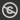Science, Maths & Technology
Free course

# Introduction to number theory## Course reviews

This free course, Introduction to number theory, is a branch of mathematics concerned with the properties of integers. Section 1 introduces Euclid’s algorithm, which is used to find the HCF of two integers, and the idea of congruences, mathematical statements used to compare remainders when two integers are each divided by another integer. Section 2 introduces modular arithmetic, in which the usual arithmetic operations are applied to congruences. Section 3 introduces multiplicative inverses, which provide a method for division in modular arithmetic, and their use in solving linear congruences which are used in cryptography for disguising information or ciphers. Please note: a Statement of Participation is not issued for this course.

## Course learning outcomes

After studying this course, you should be able to:

• find quotients and remainders from integer division
• apply Euclid's algorithm and backwards substitution
• understand the definitions of congruences, residue classes and least residues
• add and subtract integers, modulo n, multiply integers and calculate powers, modulo n
• determine multiplicative inverses, modulo n and use to solve linear congruences.

First Published: 27/09/2017

Updated: 03/05/2019

Skip Rate and Review